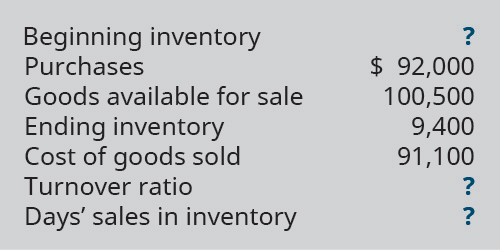# Complete the missing pieces of McCarthy Company’s inventory calculations and ratios.FindFindarrow_forward

### Principles of Accounting Volume 1

19th Edition
OpenStax
Publisher: OpenStax College
ISBN: 9781947172685

#### Solutions

Chapter
SectionFindFindarrow_forward

### Principles of Accounting Volume 1

19th Edition
OpenStax
Publisher: OpenStax College
ISBN: 9781947172685
Chapter 10, Problem 17EA
Textbook Problem
1 views

## Complete the missing pieces of McCarthy Company’s inventory calculations and ratios.To determine

Concept introduction:

Inventory Turnover Ratio:

It measures the efficiency of the firm in converting its inventory into sales. It is usually calculated by dividing the Cost of goods sold by the Average inventory. The formula of the Inventory Turnover Ratio is as follows:

Inventory Turnover Ratio=Cost of goods soldAverage inventory

Note: Average inventory is calculated with the help of following formula:

Average inventory=(Beginning inventory + Ending inventory)2

Day’s sales in inventory:

Days sales in inventory represent the number of days the inventory waits for the sale. It is calculated using the following formula:

Day Sales in Inventory =  Inventory ×365Cost of Goods Sold

To calculate:

The missing values

### Explanation of Solution

The missing values are calculated as follows:

 Beginning Inventory (A) $8,500 (100500-92000) Purchases (B)$ 92,000 Goods Available for Sale (C) = (A+B) \$ 100,500

### Still sussing out bartleby?

Check out a sample textbook solution.

See a sample solution

#### The Solution to Your Study Problems

Bartleby provides explanations to thousands of textbook problems written by our experts, many with advanced degrees!

Get Started

Find more solutions based on key concepts
How does targeted profit enter into the break-even units equation?

Managerial Accounting: The Cornerstone of Business Decision-Making

Why must employers maintain employees individual earnings records?

College Accounting (Book Only): A Career Approach

Describe the major types of adverting by purpose.

Foundations of Business (MindTap Course List)

If you were the CFO of a company that had to decide on hundreds of potential projects every year, would you wan...

Fundamentals of Financial Management, Concise Edition (with Thomson ONE - Business School Edition, 1 term (6 months) Printed Access Card) (MindTap Course List)